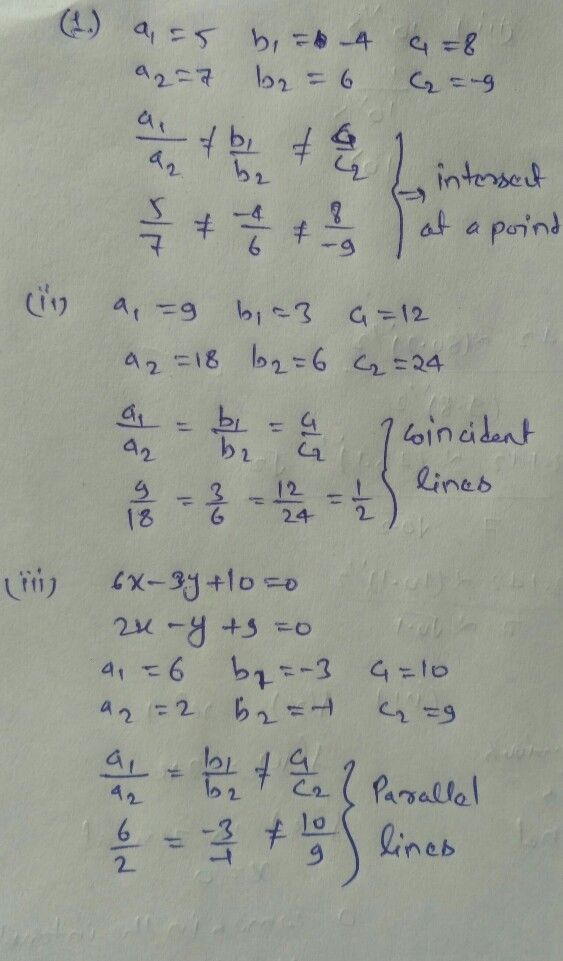Symbol
ProblemOn comparing the ratios $\dfrac {a_{1}} {a_{2}},\dfrac {b_{1}} {b_{2}}$ and $\dfrac {c_{1}} {c_{2}}$ find out whether the lines representing the following pairs of linear equations intersect at a point, are parallel or $coincident$ $\left(1\right)$ $5x-4y+8=0$ $\left(i\right)$ $9x+3y+12=0$ $7x+6y-9=0$ $18x+6y+24=0$ $\left(1\right)$ $6x-3y+10=0$ $2x-y+9=0$
10th-13th grade
Algebra
Search count: 105
SolutionQanda teacher - smyadavStudent
thanks mam
now i undarstand